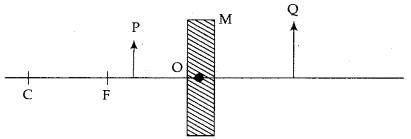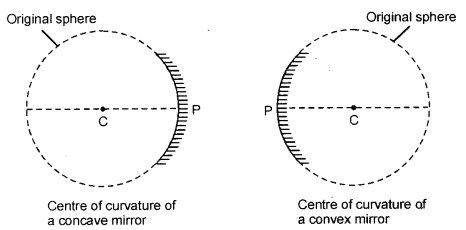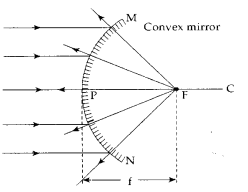# (a) Define the following terms in the context of spherical mirrors: (i) Pole

(a) Define the following terms in the context of spherical mirrors:

1. Pole
2. Centre of curvature
3. Principal axis
4. Principal focus

(b) Draw ray diagrams to show the principal focus of a :

1. Concave mirror
2. Convex mirror

(c ) Consider the following diagram in which M is a mirror and P is an object and Q is itsState the type of the mirror M and one characteristic property of the image Q.

(a)1. Pole: The middle point of the reflecting surface of a spherical mirror is called pole.
The letter P represents pole, MP = M’P.
2. Centre of curvature: It is the centre of the sphere of glass of which the mirror is a part. The letter C represents the centre of curvature.
3. Principal axis of a spherical mirror is the straight line joining the centre of curvature and pole of the mirror.
4. Principal focus: The mid-point of CP is called focus (F). It is the point on the principal axis of a spherical mirror where all incident rays parallel to the principal axis meet or appear to diverge after reflection.

(b) Ray diagrams of principal focus:

1. Concave mirror : In a concave mirror the reflected rays of incident rays parallel to principal axis actually pass through the focus (F). Thus, concave mirror has a real principal focus.2. Convex mirror : In a convex mirror the reflected rays do not actually pass through the focus (F). Thus, a convex mirror has a virtual principal focus situated behind the mirror.(c ) The mirror used in the given diagram is a concave spherical mirror. Image formed (Q) is virtual and magnified.